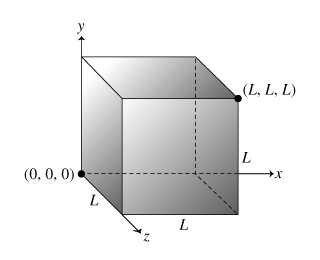# Problem: A cube has one corner at the origin and the opposite cornerat the point (L,L,L). The sides of the cube are parallel to the coordinate planes. The electric field in and around the cube is given by E = (a + bx) x̂ + c ŷ.Find the total electric flux ΦE through the surface of the cube. Express your answer in terms of a,b,c and L. What is the net charge q inside the cube? Express your answer in terms of a, b, c, L, and ϵ0.

###### FREE Expert Solution

Electric flux,

$\overline{){{\mathbf{\Phi }}}_{{\mathbf{E}}}{\mathbf{=}}\stackrel{\mathbf{⇀}}{\mathbf{E}}{\mathbf{·}}\stackrel{\mathbf{⇀}}{\mathbf{A}}}$

Gauss' law:

$\overline{){{\mathbf{\Phi }}}_{{\mathbf{E}}}{\mathbf{=}}\frac{{\mathbf{q}}_{\mathbf{e}\mathbf{n}\mathbf{c}}}{{\mathbit{ϵ}}_{\mathbf{0}}}}$

(a)

Flux through right side

x = L

E = (a + bL) + c

Aright = L2

ΦE,right = ((a + bL) + c )•(L2 ) = (a + bL)(L2 + 0) = aL2 + bL3

Flux through left side,

x = 0 - origin

E = (a + b•0) + c

Aleft = −L2

ΦE,letf = ((a + b•0)   + c )•(−L2 ) = (a)(L2 + 0) = - aL2

Flux through front side,

x = L

E = (a + bL)   + c

Afront = L2

ΦE,front = ((a + bL) + c )•(L2 ) = (0 + 0 + 0) = 0

91% (162 ratings)###### Problem Details

A cube has one corner at the origin and the opposite cornerat the point (L,L,L). The sides of the cube are parallel to the coordinate planes. The electric field in and around the cube is given by E = (a + bx) + c .Find the total electric flux ΦE through the surface of the cube. Express your answer in terms of a,b,c and L.

What is the net charge q inside the cube? Express your answer in terms of a, b, c, L, and ϵ0.

Frequently Asked Questions

What scientific concept do you need to know in order to solve this problem?

Our tutors have indicated that to solve this problem you will need to apply the Gauss' Law concept. You can view video lessons to learn Gauss' Law. Or if you need more Gauss' Law practice, you can also practice Gauss' Law practice problems.

What professor is this problem relevant for?

Based on our data, we think this problem is relevant for Professor Jerousek's class at UCF.